# Trigonometric Ratios

Trigonometry is the study of triangles, their measurements, and calculations based on these measurements. The right triangle has a special place in trigonometry because of its interesting characteristics. As previously proven, in a right triangle, the square of the length of the hypotenuse is equal to the sum of the squares of its sides. This leads to some special ratios like sine and cosine, which are used extensively in such fields as science, engineering, and architecture.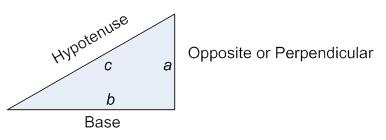# Trig Functions

Sine: In a right triangle, the ratio of the length of the opposite side to its hypotenuse (for a given angle θ or theta) is a constant and is called sinθ or the sine of the angle θ. Its inverse trig function is cosecant, denoted cscθ.
sinθ=OppositeHypotenuse cscθ=1sinθ=HypotenuseOpposite
Ex: The sine of an angle in a right triangle can also be a measure of steepness. Imagine a road that uniformly rises 6 feet in height for every 100 ft in length, as shown below. The steepness of the road is then 6100. Coincidentally, the measure of steepness is important in road construction; any value greater than 6100 is usually avoided for safety reasons.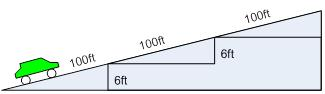Cosine: In a right triangle, the ratio of the length of its adjacent side or base to its hypotenuse is a constant for any angle θ, and is called cosθ or the cosine of the angle θ. Its inverse trig function is secant, denoted secθ.
cosθ=AdjacentHypotenuse secθ=1cosθ=HypotenuseAdjacent
Tangent: In a right triangle, the ratio of the length of its opposite side to its adjacent side or base is a constant for any angle θ, and is called tanθ or the tangent of the angle θ. Its inverse trig function is cotangent, denoted cotθ.
tanθ=OppositeAdjacent cotθ=1tanθ=AdjacentOpposite

Note

Notes

# Inverse Trig Functions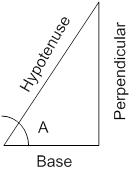Sine, cosine, and tangent give the ratios of sides in a right triangle for a given angle. But what if you know the ratio and want to find the measure of the angle? Inverse trigonometric functions solve this problem by giving an angle in a right triangle from a given ratio.
Arcsine: Arcsine gives the angle θ given the sine ratio. It is denoted arcsinx or sin-1x. In other words, when the ratio of the opposite side to the hypotenuse is given, the corresponding measure of ∠A (in the figure) is given by the arcsine of the opposite over the hypotenuse.

sinθ=OppositeHypotenuse θ=sin-1OppositeHypotenuse

Arccosine: Arccosine gives the angle θ given the cosine ratio. It is denoted arccosx or cos-1x. In other words, when the ratio of the adjacent side to the hypotenuse is given, the corresponding measure of ∠A (in the figure) is given by the arccosine of the adjacent side to the base.
cosθ=AdjacentHypotenuse θ=cos-1AdjacentHypotenuse
Arctangent: Arctangent gives the angle θ given the cosine ratio. It is denoted arccosx or cos-1x. In other words, when the ratio of the opposite side to the adjacent side is given, the corresponding measure of ∠A is given by the arccosine of the opposite side to the adjacent side.
tanθ=OppositeAdjacent θ=tan-1OppositeAdjacent

Note

Notes

# Complimentary Relationships of Trig Ratios

In a right triangle, the two angles opposite to the base and the perpendicular are complimentary. For example, in Fig 1.2.1, the angles α and β satisfy α+β=90°=π2 radians.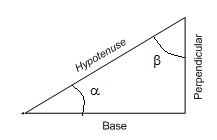Fig 1.2.1: Angles α and β are complementary
The nature of the trigonometric functions implies that the following statements are true:
cosα=sinπ2-α=sinβ
cscα=secπ2-α=secβ
cotα=tanπ2-α=tanβ

Note

Notes

# The Unit Circle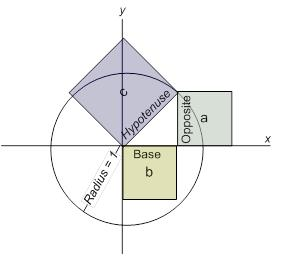A perpendicular drawn from the perimeter of a circle of radius 1 to the x-axis makes a right triangle, as shown in Fig 2.1. This circle is known as the unit circle. From this triangle, squares of trigonometric ratios can be obtained using the Pythagorean Theorem.

Fig 2.1 Squares of the sides of a right triangle formed on the unit circle.

With reference to Fig 2.1, we can simplify the trigonometric ratios by substituting 1 (the radius of the circle) as the length of the hypotenuse.
sinθ=Opposite=a
cosθ=Base=b
Using the Pythagorean Theorem, we can obtain the following important identities:
sin2θ+cos2θ=1
sin2θ=1-cos2θ or sinθ=1-cos2θ
cos2θ=1-sin2θ or cosθ=1-sin2θ
tanθ=sinθcosθ

Note

Notes

# Worksheet: Computing Sine and Cosine Using a Circle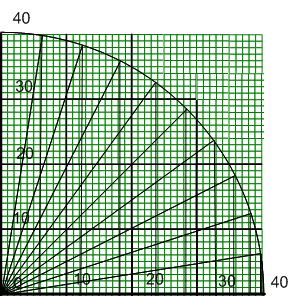While scientific calculators are able to determine sine and cosine automatically, it’s also possible to compute the ratio graphically from the triangles formed in Fig 1.2.3. Fill out the entries in the table to compute the sine and cosine of various values, and verify these values with a calculator.
Note: The hypotenuse in all of the triangles is 40 units, and the quarter circle (90°) is divided into 10 angles. a=opposite, b=adjacent, and c=hypotenuse.

Fig 2.2. Graphical determination of sine and cosine

θ
c
a
b
sinθ
cosθ
tanθ
sinθ from calculator
cosθ from calculator
9

18

27

36

45

54

63

72

81

90

Note

Notes

# Trig Ruler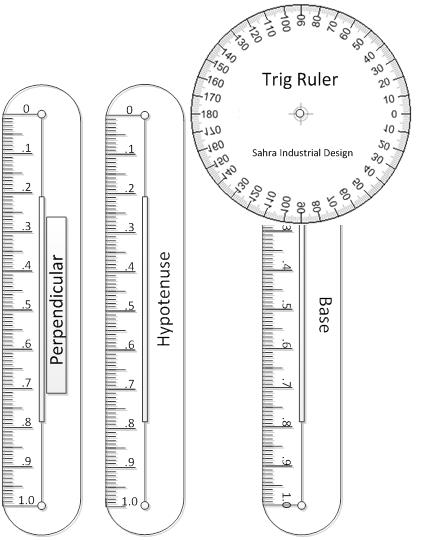A trigonometric ruler can be constructed that shows the values of the trigonometric functions without the use of a calculator. The ruler consists of three scales, labeled Hypotenuse, Base, and Perpendicular, and a circular disc measuring angles around a circle from 0° to 360°, as shown in Fig 1.2.4. The length of each scale is 1 unit, but it is also divided into 100 smaller units.
To assemble the trig ruler, cut out the scales and the circular disc and attach as shown in Fig 1.2.5. (Note: the perpendicular is suspended from the other end of the hypotenuse.)

Fig 2.3. Trig Ruler scales and circular disc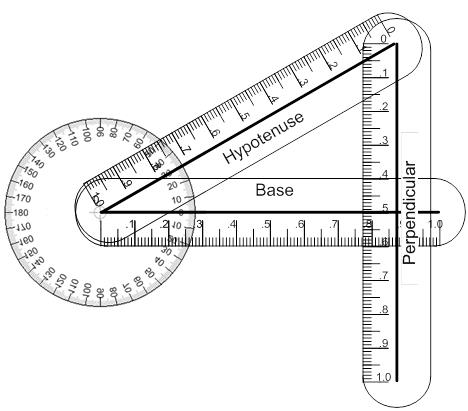Fig 2.4. Trig Ruler assembly

Note

Notes

# Using the Trig Ruler

You can calculate the height of an object (such as a building or a tree) and its distance from you with the help of the Trig Ruler by simply measuring the angle of your line of sight.
To find out how far away an object is, stand at a distance and align the Trig Ruler such that the base is horizontal and parallel to the ground and the hypotenuse is aligned toward the bottom of the object, as shown in Fig 1.2.6. By doing this, you are creating two similar triangles. Using the fact that the sides of similar triangles are proportional, you can find the object’s distance x from you.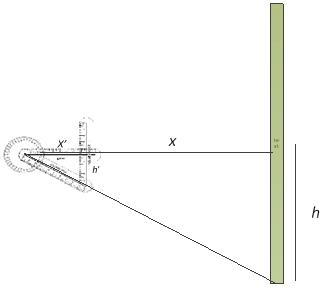Note: You are looking at the object from your height, so the height h is same as your height. The height h' is the reading from the Trig Ruler’s Perpendicular scale and the distance x’ is the reading from the Trig Ruler’s Base scale (where the Base and the Perpendicular intersect). x is the distance from the object to you.

This gives you your distance from the object, x, as: Fig 2.5. Using the Trig Ruler to measure distances
xh=x'h'
The height H of the object can be calculated by aligning the hypotenuse toward the top of the object and measuring the Trig Ruler’s Base and Perpendicular scales at the point where they intersect. If the base scale measures x and the perpendicular scale measures h, then the height of the object can be found by using: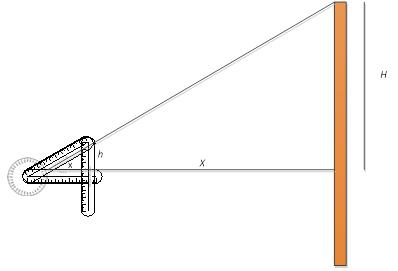Hh=Xx

Fig 2.6. Using the Trig Ruler to find the height of an object

Note

Notes